# Hemispherical Shell under Concentrated LoadsThe purpose of this test is to check shell elements with double curvature. You will use 2D meshes.Reference:

MAC NEAL R.H., HARDER R.L., A Proposed Standard Set of Problems to Test Finite Element Accuracy, Finite Element Design, Vol.1, pp.3-20, 1985.

##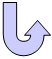Specifications

### Geometry Specifications

 Radius: R = 10 m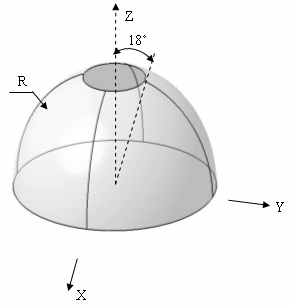Angle: θ = 18 deg Thickness: th=0.04 m Equation: x2 + y2 + z2 = 100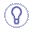For symmetry reasons, only a quarter of the hemisphere is modeled. The given results are the same for a quarter or for the whole hemisphere.

### Analysis Specifications

 Young Modulus (material): E = 68.25 MPa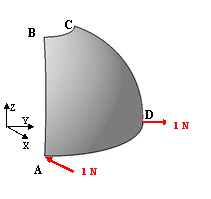Poisson's Ratio (material): ν= 0.3 Mesh Specifications: Imposed number of elements on AB, CD and DA edges  Imposed middle point for triangle mesh See the values in the table of results. Restraints (User-defined): At point A: Tz=0 On edge AB: Ty=Rx=Rz=0 On edge CD: Tx=Ry=Rz=0 Loads (Distributed force): F=1 (outward at A, inward at D)

##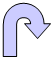Results

• The analytical solution is:
At point A, x-displacement is 94 mm.

• The results correspond to the x-displacements at point A.
The table below presents the analysis results.
The normalized results (computed results divided by analytical solution) are listed.

 Nodes Values Linear triangle (TR3) Parabolic triangle (TR6) Linear quadrangle (QD4) Parabolic quadrangle (QD8)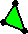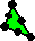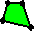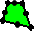Computed results [mm] Normalized results Computed results [mm] Normalized results Computed results [mm] Normalized results Computed results [mm] Normalized results 3 x 3 105.9 1.127 99.97 1.063 71.17 0.757 23.00 0.245 5 x 5 98.02 1.043 100.46 1.069 96.05 1.022 79.25 0.843 7 x 7 95.76 1.019 101.95 1.085 96.47 1.026 94.75 1.008 9 x 9 94.85 1.009 102.26 1.088 95.48 1.016 95.52 1.016 11 x 11 94.97 1.010 103.75 1.104 94.80 1.009 94.52 1.006 13 x 13 93.32 0.993 101.87 1.084 93.90 0.999 93.68 0.997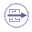To Perform the Test:

The hemispherical_shell_concentrated_loads_13nodes_tr6.CATAnalysis document presents a complete analysis of this case, computed with a mesh formed of parabolic triangle elements (TR6).

The hemispherical_shell_concentrated_loads_13nodes_qd8.CATAnalysis document presents a complete analysis of this case, computed with a mesh formed of parabolic quadrangle elements (QD8).

To compute the case with other types of elements and number of nodes, proceed as follow:

1. Open one of the CATAnalysis documents.

2. Enter the Advanced Meshing Tools workbench.

3. In the specification tree, double-click on the mesh.

The Global Parameters dialog box appears.

4. Select the Linear element type.

5. Compute the case in the Generative Structural Analysis workbench.